﻿ 16074310 余冲冲 犯罪模型 - 计算数学达人 - 专，学者，数值代数，微分方程数值解

# 一、问题分析

1)         进行信息的查询和搜集。由于本题中不存在完全有效的数据，我们必须寻找连环犯罪地点的资料。（本次实验是在网上查找的犯罪信息）

2)         建立Rossmo模型定位连环杀手的居住地点，运用该方案对居住地点进行预测，之后对方案和最后的结果进行评价和改进。

3)         预测下一个案发地点的可能地理位置和可能的案发时间。在这一步中，我们可以使用步骤2或原始数据的结果并且对预测的可靠性必须要有相关数据验证。

1、假设罪犯居住地不会改变且只有一个居住地。

2、假设一般罪犯作案范围不会很大，基本控制在一两个城市之间。

3、假设罪犯活动不受地势和交通影响。

4、假设连环案作案是单人行动，不考虑团伙作案。

5、假设罪犯作案地点基本选择在自己的居住地附近。

# 三、模型Rossmo公式预测犯罪人居住地模型

Rossmo公式构造的模型是一种被普遍用来预测罪犯居住地的方法。首先将需要处理的地区划分为 I x j 个小区域，运用Rossmo公式求得罪犯居住地在第ij个小区域的可能性，以此来确定罪犯的最可能的居住地范围。

Rossmo 公式：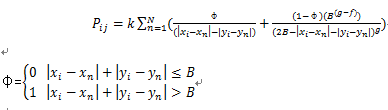是居住地在区域ij的可能性；

N       案件发生总数；是区域ij的坐标；,是第n个犯罪地点的坐标；

B      是缓冲区域的半径，即最匹配圆半径；

φ      是一个权重系数；

k      是一个经验决定的常数；

fg   是一个经验决定的指数，用来调节距离影响；

r      是最匹配圆半径。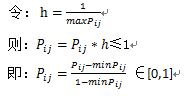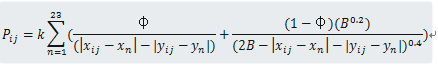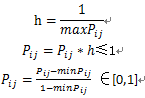23次作案地点的坐标：

 作案顺序1 0.8832 0.4020 2 0.7994 0.5721 3 0.7423 0.3525 4 0.8083 0.6609 5 1.1192 0.3386 6 1.1243 0.3069 7 1.1675 0.3462 8 1.1713 0.3360 9 0.9009 0.4236 10 1.1294 0.3259 11 0.8933 0.3767 12 0.1179 0.0353 13 1.1142 0.3195 14 0.8806 0.4084 15 0.7639 0.1711 16 0.1661 0.0556 17 1.0570 0.4109 18 0.7144 0.3081 19 0.8857 0.3881 20 1.0875 0.3538 21 1.1002 0.3272 22 0.7613 0.2244 23 1.0177 0.3525

# 五、根据处理后的矩阵通过malab画出概率分布图: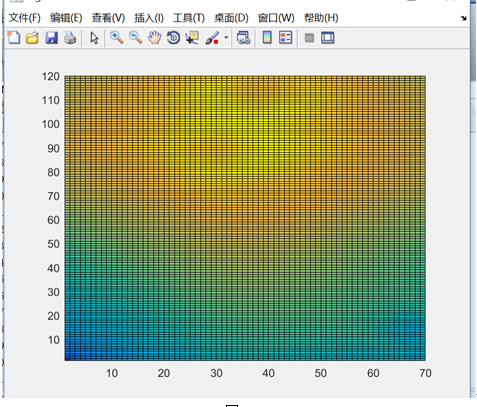# 实现代码：A=[ 0.8832 0.4020

0.7994 0.5721

0.7423 0.3525

0.8083 0.6609

1.1192 0.3386

1.1243 0.3069

1.1675 0.3462

1.1713 0.3360

0.9009 0.4236

1.1294 0.3259

0.8933 0.3767

0.1179 0.0353

1.1142 0.3195

0.8806 0.4084

0.7639 0.1711

0.1661 0.0556

1.0570 0.4109

0.7144 0.3081

0.8857 0.3881

1.0875 0.3538

1.1002 0.3272

0.7613 0.2244

1.0177 0.3525

];

x=A(:,1);y=A(:,2);B=0.1141;C=zeros(1,2);Pi=[];f=0.2;g=0.4;hang=0;Pij=0;

for a=0.005:0.01:1.2

hang=hang+1;lie=0;

for b=0.695:-0.01:0.005

P=0;lie=lie+1;

for i=1:23

q=0;

if abs(a-x(i))+abs(b-y(i))>B

q=1;

end

P=P+1/31.0033*(q/(abs(a-x(i))+abs(b-y(i)))^f+(1-q)*B^(g-f)/(2*B-abs(a-x(i))-abs(b-y(i)))^g);  %Rossmo公式

end

if P>Pij

Pij=P;

C(1)=a;C(2)=b;

end

Pi(hang,lie)=P;%求概率分布矩阵?

end

end

Pij

C

Pi=Pi-min(min(Pi));

Pi=Pi/max(max(Pi))*255;

% Pi=abs(Pi-255);%获取彩色概率分布图时需注释掉

Pi=Pi.^0.4;

H=pcolor(Pi)

% colormap(gray(256))%获取彩色概率分布图时需注释掉